# 图像处理中正交变换的目的

## 《数字图像处理》第5讲——傅里叶变换

2018-12-05 10:54:27 weixin_40851250 阅读数 8002
• ###### 人与世界的交互、视频信息的意义

通过本系列课程，观众有望理解视频压缩编码技术的整体发展，精通H.264视频编码技术的框架与细节，为进一步研究H.265/HEVC编码标准、音视频流媒体、视频直播点播等技术奠定坚实的基础。本系列课程适合多媒体方向的...

34609人学习 殷汶杰
免费试看

①傅里叶变换的作用

②知识储备

1、泰勒级数

2、欧拉公式（真正的宇宙第一公式）

3、三角级数

4、三角函数系的正交性

③傅里叶变换对

④傅立叶变换的相关概念

⑤一维傅立叶逆变换

⑥傅立叶谱的平移

⑦傅立叶变换频谱

⑧二维Fourier变换的应用

1、Fourier变换在图像滤波中的应用

2、Fourier变换在图像压缩中的应用

3、Fourier变换在卷积中的应用

## 楔子

https://zhuanlan.zhihu.com/p/19763358

http://k.sina.com.cn/article_6385529085_17c9b70fd001006gjp.html?from=science

## ①傅里叶变换的作用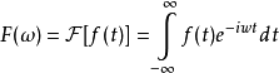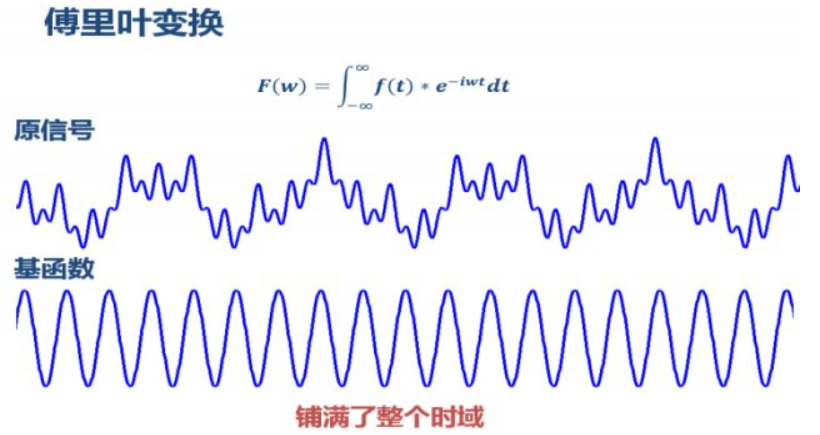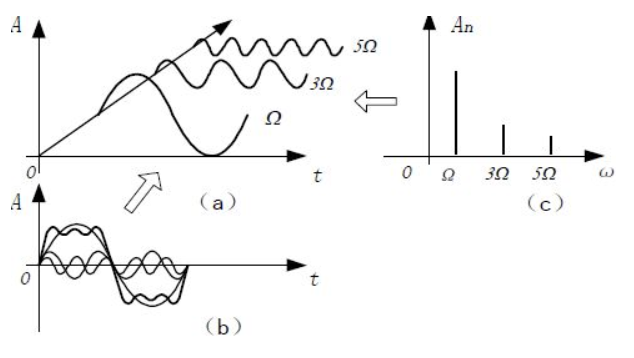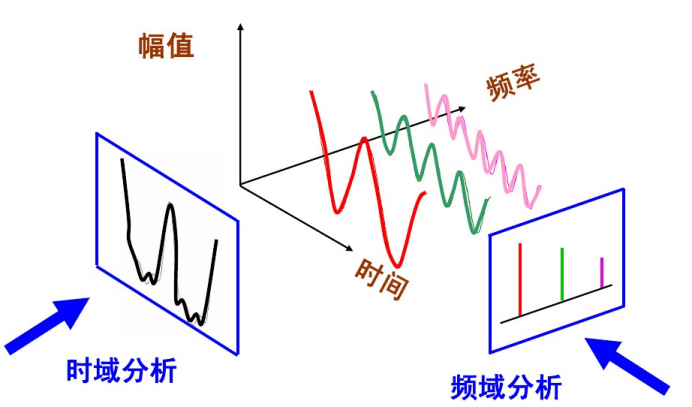## ②知识储备

### 1、泰勒级数

（https://www.zhihu.com/question/21149770）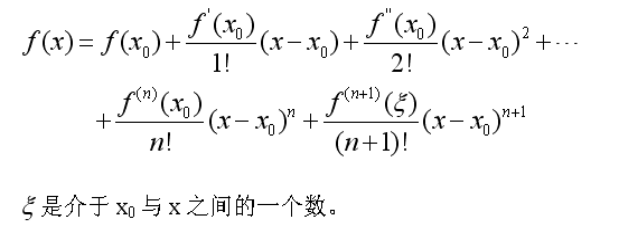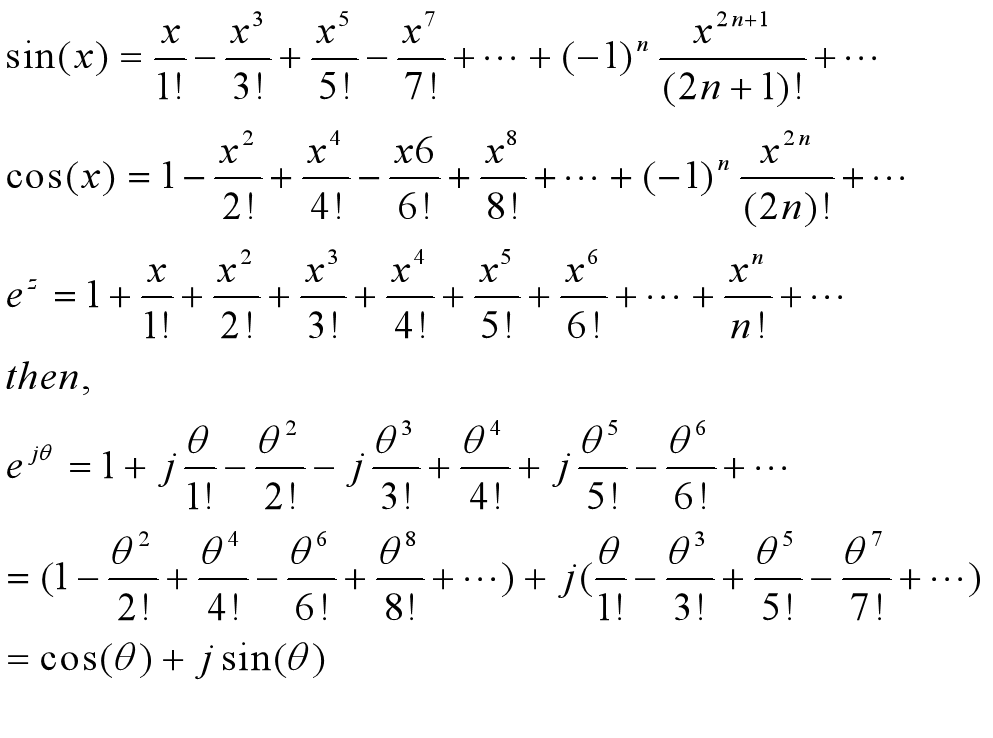### 2、欧拉公式（真正的宇宙第一公式）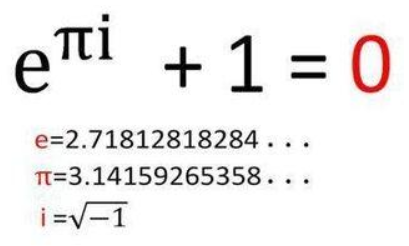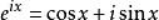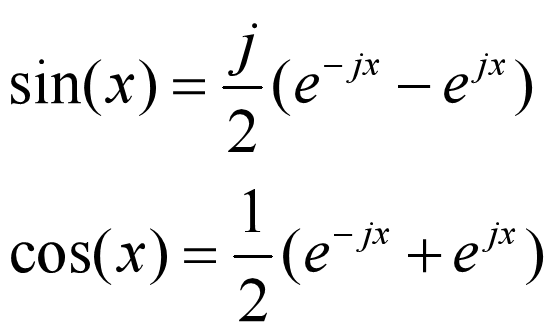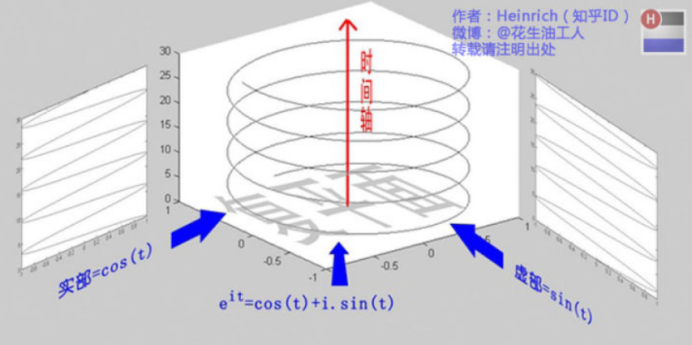### 3、三角级数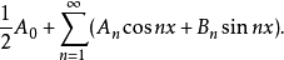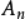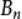具有以下形式时，该级数称为傅立叶级数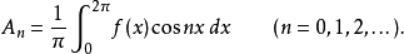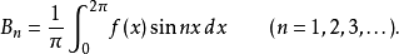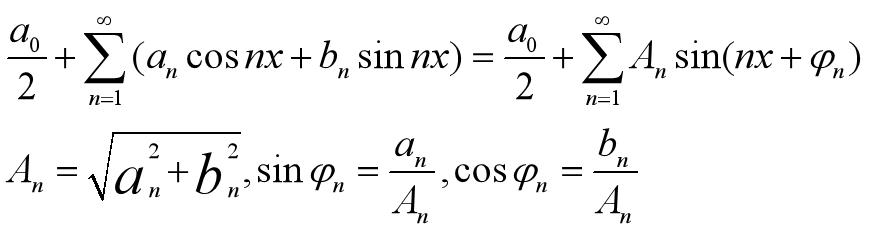### 4、三角函数系的正交性

1）其中任意两个不同的函数之积在[-π，π]上的积分等于0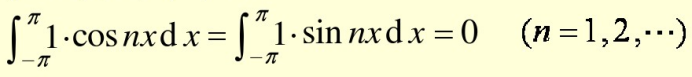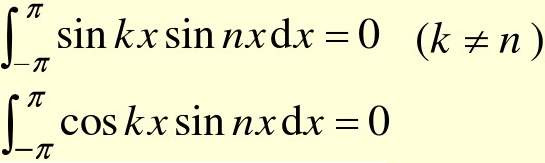2）两个相同的函数的乘积在[-π，π]上的积分不等于0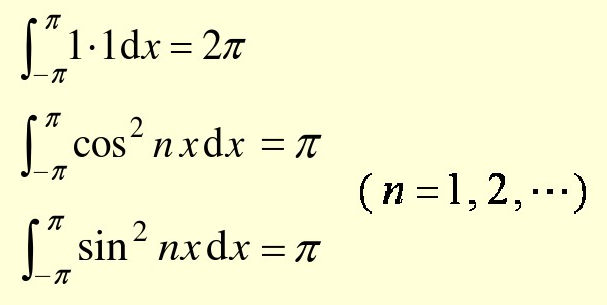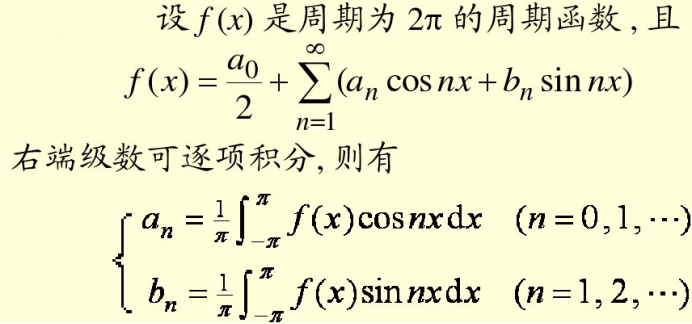## ③傅里叶变换对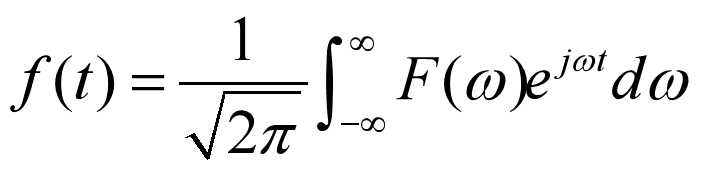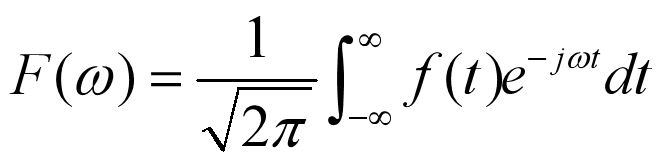## ④傅立叶变换的相关概念

###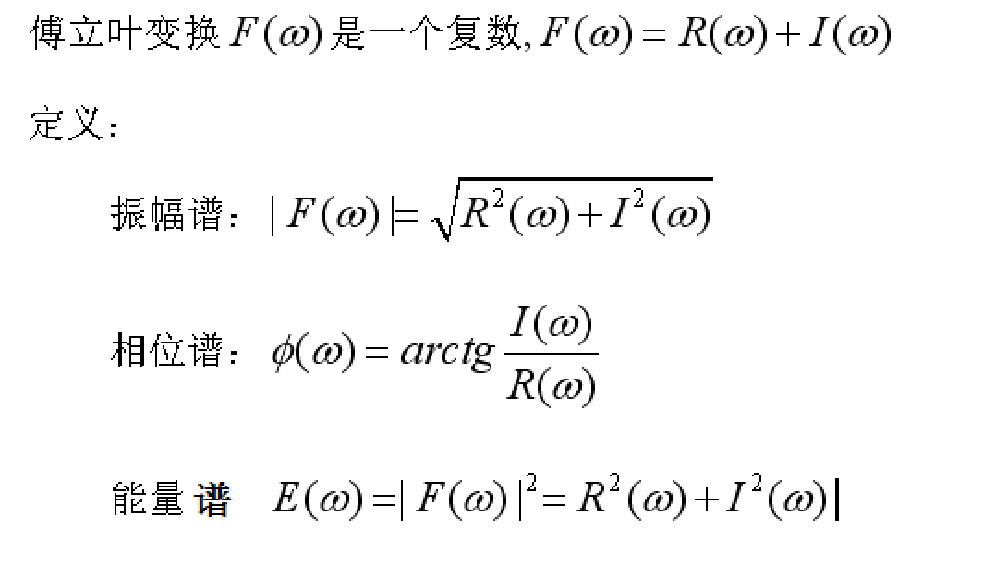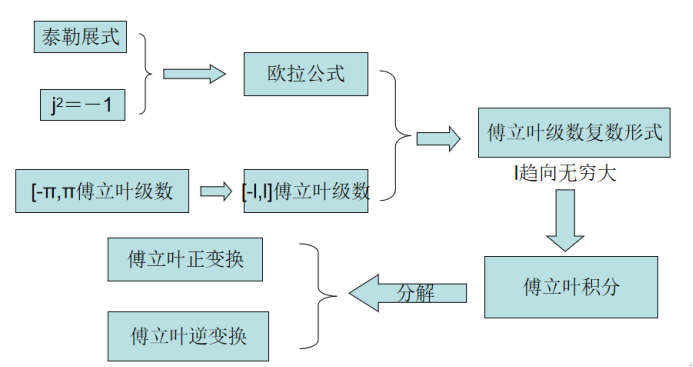## ⑤一维傅立叶逆变换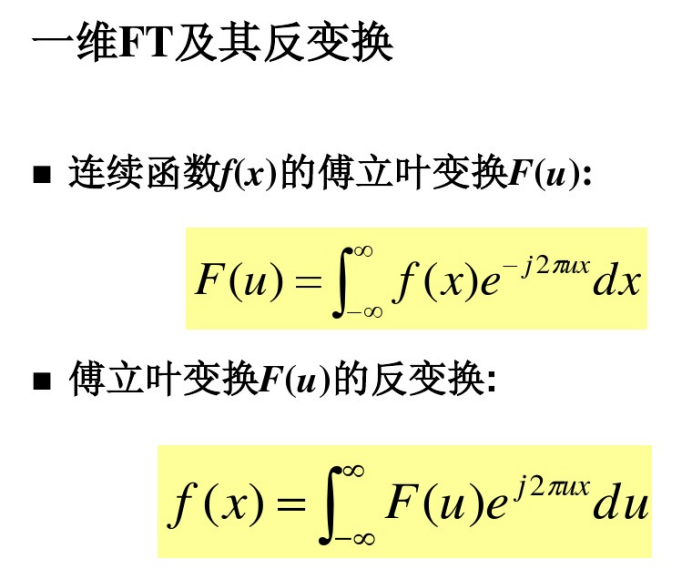## ⑥傅立叶谱的平移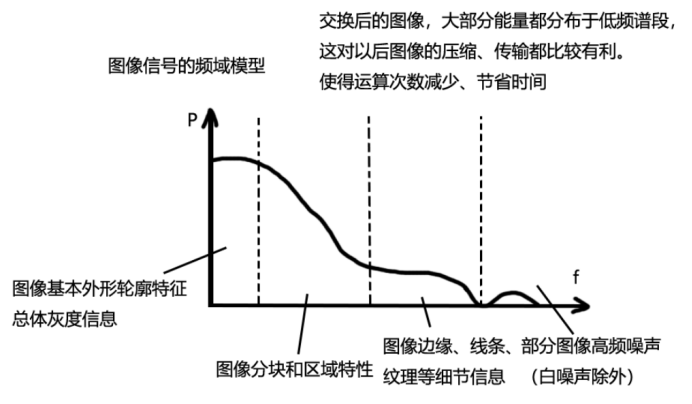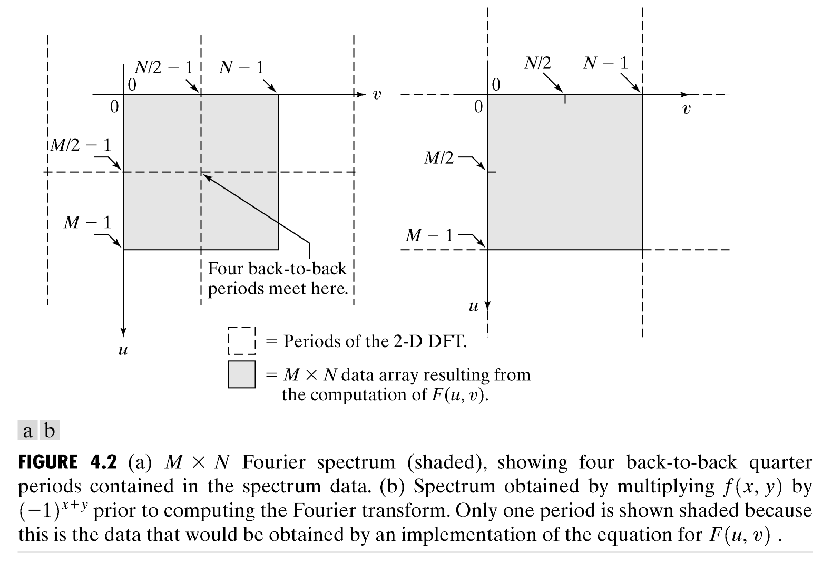## ⑦傅立叶变换频谱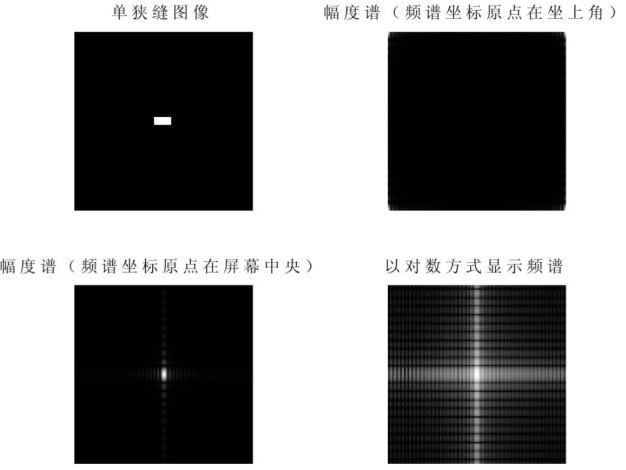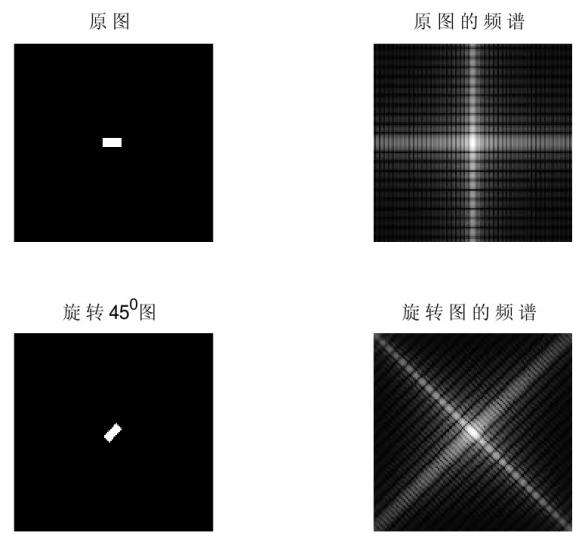## ⑧二维Fourier变换的应用

### 3、Fourier变换在卷积中的应用

从前面的图像处理算法中知道，如果抽象来看，其实都可以认为是图像信息经过了滤波器的滤波（如：平滑滤波、锐化滤波等 ）。 如果滤波器的结构比较复杂时，直接进行时域中的卷积运算是不可思议的。

https://zhuanlan.zhihu.com/p/19763358

http://k.sina.com.cn/article_6385529085_17c9b70fd001006gjp.html?from=science

## 2_图像处理中正交变换的目的是什么？图像变换主要用于那些方面？

2019-03-10 09:33:00 weixin_30570101 阅读数 383
• ###### 人与世界的交互、视频信息的意义

通过本系列课程，观众有望理解视频压缩编码技术的整体发展，精通H.264视频编码技术的框架与细节，为进一步研究H.265/HEVC编码标准、音视频流媒体、视频直播点播等技术奠定坚实的基础。本系列课程适合多媒体方向的...

34609人学习 殷汶杰
免费试看

## 数字图像处理研究的主要内容

2017-09-25 09:41:08 VonSdite 阅读数 5323
• ###### 人与世界的交互、视频信息的意义

通过本系列课程，观众有望理解视频压缩编码技术的整体发展，精通H.264视频编码技术的框架与细节，为进一步研究H.265/HEVC编码标准、音视频流媒体、视频直播点播等技术奠定坚实的基础。本系列课程适合多媒体方向的...

34609人学习 殷汶杰
免费试看

# 数字图像处理研究的主要内容

• ``````    数字图像处理是指将一幅图像变成另一幅经过修改（改进）的图像，是一个由图像到图像的过程。其主要研究内容如下：
``````
• 图像变换
• 图像增强
• 图像恢复
• 图像分割
• 数学形态学
• 图像编码与压缩

# 图像变换

• 灰度变换：包括灰度线性变换、阈值变换、窗口变换、灰度拉伸与均衡等。灰度变换目的在于：控制图像灰度直方图的分布，改善输出的图像。

• 几何变换：图像平移、镜像、转置、缩放和旋转等。对于数字化标定、图像配准、校正、投影及特殊视觉特技效果的生成十分有用。

• 正交变换：包括傅立叶变换、离散余弦变换、Walsh变换、小波变换等。正交变换是将图像的二位数据矩阵看作特殊的二维信号，并将其变换到频域，从而加强对图像信息的辨识与理解。广泛运用于图像特征提取、图像增强、图像压缩和图像识别领域。

# 图像增强

• ``````  空域滤波增强和频域滤波增强技术，具体方法如**图像模板操作、平滑、锐化、中值滤波、低/高通滤波、伪色彩增强等。**
``````
• 主要目的是根据任务目标突出图像中感兴趣的信息，消除干扰，改善图像的视觉效果或增强便于机器识别的信息。

# 图像恢复

• ``````根据图像退化模型，消除或减轻在图象获取及传输过程中造成的图像品质下降即退化现象，恢复图像的本来面目。退化包括由成像系统光学特性造成的歧变以及噪声和相对运动造成的模糊等情况。
``````
• 运动模糊图像的恢复# 图像分割

• ``````    包括**阈值分割法、基于梯度的分割法及边界检测与跟踪**等。
``````
• 主要目的是根据图像的某些特征将图像划分为互不重叠的区域，以便于对图像中的物体或目标进行分析与识别。图像分割是图像分析和理解的基础

# 数学形态学

• ``````    形态学运算主要用来处理**二值图像**，其数学基础是集合论。常见的形态学运算有**膨胀、腐蚀、开运算、闭运算、细化**等。
``````
• 数学形态学是分析图像几何特征的有利工具。

# 图像编码与压缩

• ``````要在计算机连续显示分辨率为1280x1024的24位真彩色高质量的电视图像，按每秒30祯计算，显示1分钟需要6.6GB。可见，数字图像数据的压缩问题是多媒体技术的重要研究课题。
``````
• ``````图像编码与压缩就是对要处理的图像数据用一定的规则进行变换和组合，从而达到以尽可能小的代码来表示尽可能多的数据信息的目的，常见的压缩编码有**霍夫曼编码、游程编码、JPEG编码**等。
``````
• ``````研究压缩图像数据的方法，需要研究并利用图像的冗余特征如统计冗余、生理视觉冗余、知识冗余等。
``````

## 基于小波变换的图像去噪matlab仿真

2016-05-02 16:18:14 liaozali9022 阅读数 16383
• ###### 人与世界的交互、视频信息的意义

通过本系列课程，观众有望理解视频压缩编码技术的整体发展，精通H.264视频编码技术的框架与细节，为进一步研究H.265/HEVC编码标准、音视频流媒体、视频直播点播等技术奠定坚实的基础。本系列课程适合多媒体方向的...

34609人学习 殷汶杰
免费试看

1、概况

matlab设计流程：

与单纯运用某种自适应算法相比,基于小波分解的自适应滤波算法在收敛速度和稳定性上都有了很大的提高

2、小波变换的基本理论

示意：尺度越大，采用越大的时间窗，尺度越小，采用越短的时间窗，即尺度与频率成反比。

小波变换与傅里叶变换对比的优点：短时傅立叶分析是把一个短时间的窗函数加在信号上，再对这一部分加窗的信号做傅立叶变换，当然，假定了在短时间内信号是平稳的。然后移动窗函数，对整个时间轴上的信号做短时傅立叶分析。但是，又有问题出现了，短时傅立叶分析一旦确定了窗函数，窗函数的形状便不再改变，于是短时傅立叶分析对于信号的时频分辨率也随之确定。

小波变换的物理表现：分解的结果是产生长度减半的两个部分，一个是经低通滤波器产生的原始信号的平滑部分，另一个则是经高通滤波器产生的原始信号细节部分。

不同j所确定的频带是独立的，随j变化相互独立的频带覆盖了整个频率轴，分辨率j反应了频带的位置和带宽把信号分解到一系列相互独立的频带上分辨率j-1时的近似信号=f(x)在分辨率为j时的近似部分+细节部分

3、小波变换在matlab中的使用

[C, S] = wavedec2(x, n, wname); % 对图像进行小波分解

[C,S]的含义C=[A(n)|H(n)|D(n)|………H(1)|V(1)|D(1)]

h ,v ,d 分别反映水平、垂直、对角线方向

S(1,:)=size of approximation coefficients(n)

%读取MATLAB中的名为detfinger的图像  分辨率：512×512

subplot(1,2,1)

imshow(M);           %显示原始图像

title('原始图像')

P1=imnoise(M,'gaussian',0.02);

%加入高斯躁声,方差为0.02的高斯噪声

P2=imnoise(P1,'salt &pepper',0.02);

%同时加入高斯噪声和椒盐躁声

subplot(1,2,2)

imshow(P2);%加入椒盐躁声后显示图像

title('加入高斯椒盐躁声后');

%%对加入高斯噪声的图像进行小波分解

P2=double(P2);

[CA,CH,CV,CD]= dwt2(P2,'db1 ');

y =[CA,CH;CV,CD];

y=uint8(y);

subplot(3,1,3);

imshow(y);

title('小波分解');

4、滤波器的特性

5、如何设计和建立自适应滤波器

自适应滤波器工作原理是:系统能够按照某种算法自动调节权系数,使其实际输出和期望输出的均方误差达到最小值。

自适应滤波器的结构：有FIRIIR两种。由于IIR滤波器存在稳定性的问题,因此一般采用FIR滤波器。由于FIR滤波器横向结构的算法具有容易实现和计算量少等优点,在对收敛速度不是很快的场合,多采用FIR作为自适应滤波器结构。

LMS算法由于其具有计算量小,稳定性好,易于实现等优点,被广泛使用。

LMS算法中均方误差表示为:

（1）自适应算法中步长 μ 的选择：μ 的大小影响算法的收敛速度。  其中，为矩阵的最大特征值μ 取值小，收敛速度慢，计算工作量大，但滤波性能较好。μ 取值大，收敛速度快，计算工作量小，滤波性能较差。但 μ 大到一定值时，收敛速度变化不明显，且取值过大，会造成计算溢出.

(2)自适应滤波器阶数 的影响： N 不可以任意选取，需要根据经验加上实际仿真验证比较才能最终确定.

、参考信号d(n)、误差信号e(n)

e(n)y(n)d(n)的误差信号，根据最小均方误差算法调节自适应滤波器算法的参数，来优化滤波器结构，从而实现尽可能地保留图像固有的信息。

LMS算法最核心的思想是用平方误差代替均方误差,基本的LMS算法为:

w(k,n+ 1)=w(k,n)+ 2μe(n)x(n-k)

… ,N- 1,N为滤波器的阶数；μ为收敛因子；e(n)=d(n)-y(n)=d(n)-xT(n)w(n)=d(n)-wT(n)x(n)为误差信号；x(n-k)为输入信号；y(n)=xT(n)w(n)为输出信号

6、matlab仿真

%%对加入高斯噪声的图像进行小波分解

J=double(J);

[CA,CH,CV,CD]= dwt2(J,'db1 ');

% [c,s]=wavedec2(j,1,'haar');

y =[CA,CH;CV,CD];

y=uint8(y);

subplot(2,2,3);

imshow(y);

title('小波分解');

%xx=wiener2(CA,[3 3]); %对加噪图像进行二维自适应维纳滤波   %滤波器窗口大小

yy=wiener2(CH,[3 3]); %对加噪图像进行二维自适应维纳滤波   %滤波器窗口大小

zz=wiener2(CV,[3 3]); %对加噪图像进行二维自适应维纳滤波   %滤波器窗口大小

qq=wiener2(CD,[3 3]); %对加噪图像进行二维自适应维纳滤波   %滤波器窗口大小

%%%图像重构

XX = idwt2(CA,yy,zz,qq,'db1');

XX=uint8(XX);

subplot(2,2,4);

imshow(XX);

title('重构以后的图像');

6自适应滤波器的组合设计：

但是当信号源中混有多种干扰噪声时,单独一个滤波器不能达到抵消多种干扰噪声的要求。针对信号源中混有的多种干扰噪声问题,提出了自适应滤波器的组合设计问题。

(不选)      基于小波分解重构的自适应滤波算法比仅进行小波分解的自适应滤波算法滤波精度要稍高一些,但由于需要信号重构,算法的复杂度随之增加,因此该算法实时性相对稍差。

## [Python图像处理] 二十二.Python图像傅里叶变换原理及实现

2019-04-23 16:24:29 Eastmount 阅读数 19613
• ###### 人与世界的交互、视频信息的意义

通过本系列课程，观众有望理解视频压缩编码技术的整体发展，精通H.264视频编码技术的框架与细节，为进一步研究H.265/HEVC编码标准、音视频流媒体、视频直播点播等技术奠定坚实的基础。本系列课程适合多媒体方向的...

34609人学习 殷汶杰
免费试看

PS：请求帮忙点个Star，哈哈，第一次使用Github，以后会分享更多代码，一起加油。

1.图像傅里叶变换
2.Numpy实现傅里叶变换
3.Numpy实现傅里叶逆变换
4.OpenCV实现傅里叶变换
5.OpenCV实现傅里叶逆变换

PS：文章参考自己以前系列图像处理文章及OpenCV库函数，同时参考如下文献：
《数字图像处理》（第3版），冈萨雷斯著，阮秋琦译，电子工业出版社，2013年.
《数字图像处理学》（第3版），阮秋琦，电子工业出版社，2008年，北京.
《OpenCV3编程入门》，毛星云，冷雪飞，电子工业出版社，2015，北京.

tenderwx-数字图像处理-傅里叶变换在图像处理中的应用

# 一.图像傅里叶变换原理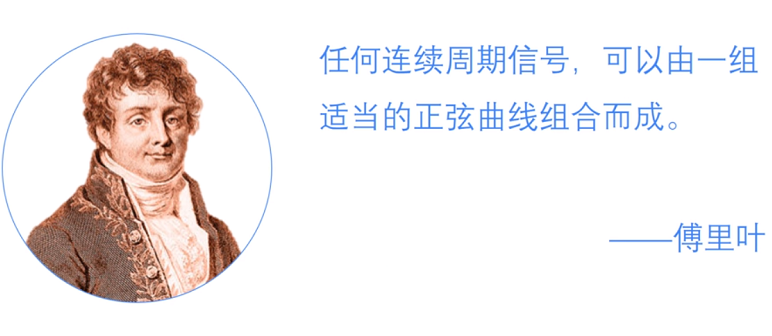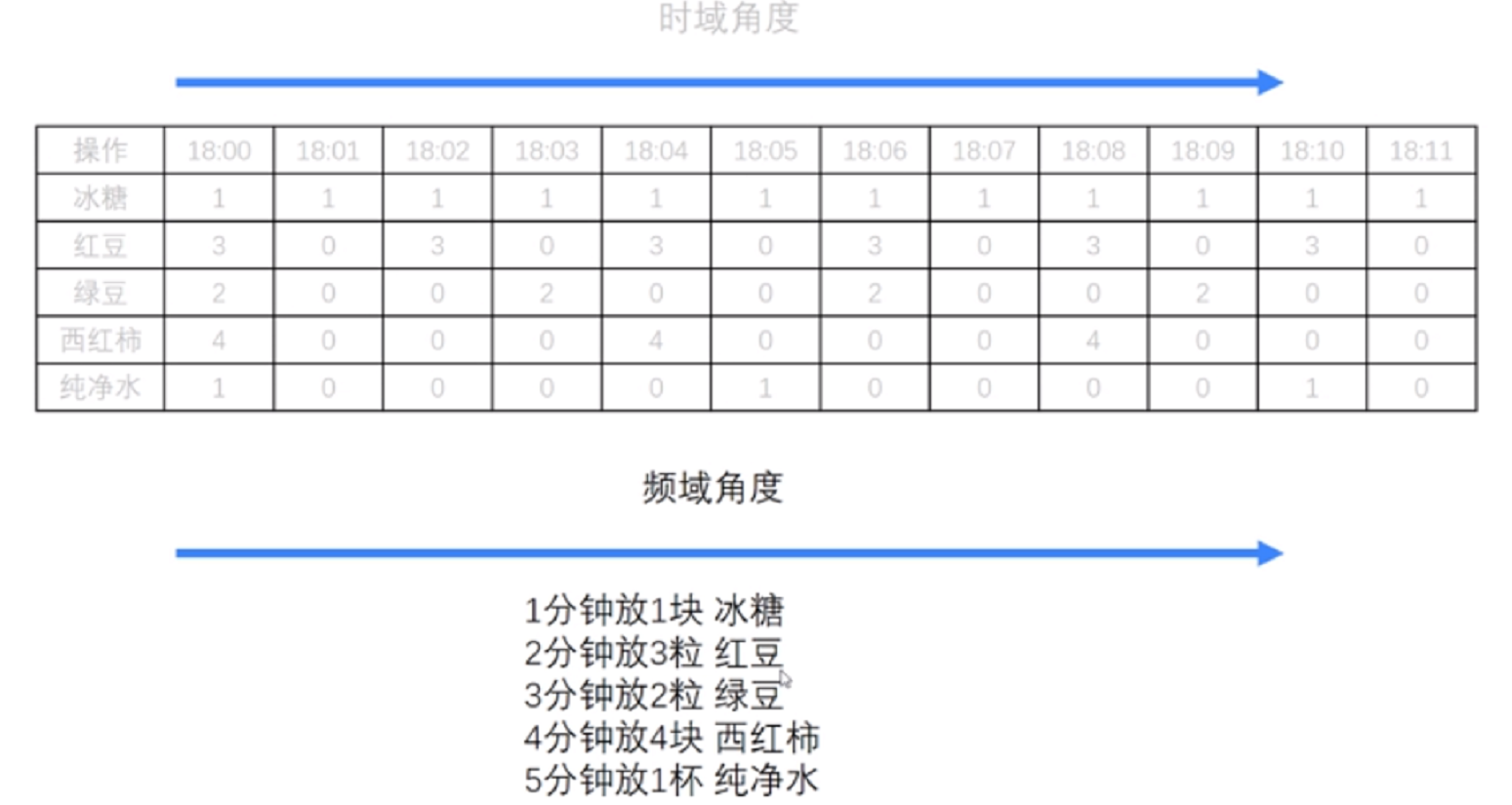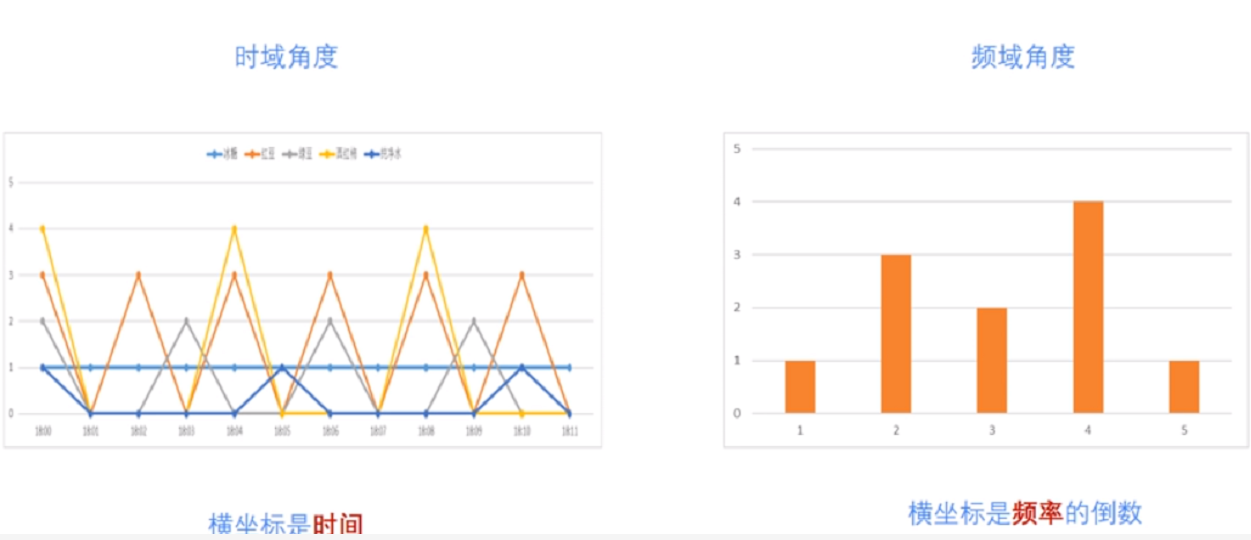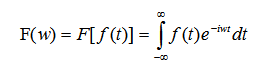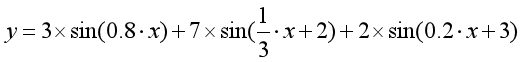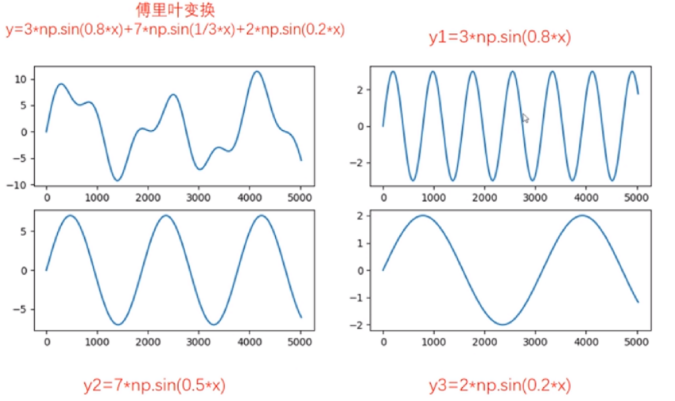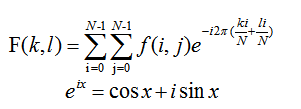# 二.Numpy实现傅里叶变换

Numpy中的 FFT包提供了函数 np.fft.fft2()可以对信号进行快速傅里叶变换，其函数原型如下所示，该输出结果是一个复数数组（Complex Ndarry）。

fft2(a, s=None, axes=(-2, -1), norm=None)

• a表示输入图像，阵列状的复杂数组
• s表示整数序列，可以决定输出数组的大小。输出可选形状（每个转换轴的长度），其中s表示轴0，s表示轴1。对应fit(x,n)函数中的n，沿着每个轴，如果给定的形状小于输入形状，则将剪切输入。如果大于则输入将用零填充。如果未给定’s’，则使用沿’axles’指定的轴的输入形状
• axes表示整数序列，用于计算FFT的可选轴。如果未给出，则使用最后两个轴。“axes”中的重复索引表示对该轴执行多次转换，一个元素序列意味着执行一维FFT
• norm包括None和ortho两个选项，规范化模式（请参见numpy.fft）。默认值为无

Numpy中的fft模块有很多函数，相关函数如下：

#计算一维傅里叶变换
numpy.fft.fft(a, n=None, axis=-1, norm=None)
#计算二维的傅里叶变换
numpy.fft.fft2(a, n=None, axis=-1, norm=None)
#计算n维的傅里叶变换
numpy.fft.fftn()
#计算n维实数的傅里叶变换
numpy.fft.rfftn()
#返回傅里叶变换的采样频率
numpy.fft.fftfreq()
#将FFT输出中的直流分量移动到频谱中央
numpy.fft.shift()

``````# -*- coding: utf-8 -*-
import cv2 as cv
import numpy as np
from matplotlib import pyplot as plt

#读取图像

#快速傅里叶变换算法得到频率分布
f = np.fft.fft2(img)

#默认结果中心点位置是在左上角,
#调用fftshift()函数转移到中间位置
fshift = np.fft.fftshift(f)

#fft结果是复数, 其绝对值结果是振幅
fimg = np.log(np.abs(fshift))

#展示结果
plt.subplot(121), plt.imshow(img, 'gray'), plt.title('Original Fourier')
plt.axis('off')
plt.subplot(122), plt.imshow(fimg, 'gray'), plt.title('Fourier Fourier')
plt.axis('off')
plt.show()
``````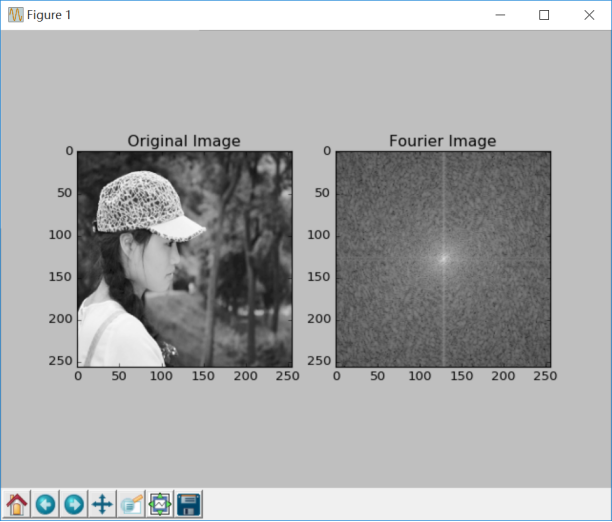# 三.Numpy实现傅里叶逆变换

#实现图像逆傅里叶变换，返回一个复数数组
numpy.fft.ifft2(a, n=None, axis=-1, norm=None)
#fftshit()函数的逆函数，它将频谱图像的中心低频部分移动至左上角
numpy.fft.fftshift()
#将复数转换为0至255范围
iimg = numpy.abs(逆傅里叶变换结果)

``````# -*- coding: utf-8 -*-
import cv2 as cv
import numpy as np
from matplotlib import pyplot as plt

#读取图像

#傅里叶变换
f = np.fft.fft2(img)
fshift = np.fft.fftshift(f)
res = np.log(np.abs(fshift))

#傅里叶逆变换
ishift = np.fft.ifftshift(fshift)
iimg = np.fft.ifft2(ishift)
iimg = np.abs(iimg)

#展示结果
plt.subplot(131), plt.imshow(img, 'gray'), plt.title('Original Image')
plt.axis('off')
plt.subplot(132), plt.imshow(res, 'gray'), plt.title('Fourier Image')
plt.axis('off')
plt.subplot(133), plt.imshow(iimg, 'gray'), plt.title('Inverse Fourier Image')
plt.axis('off')
plt.show()
``````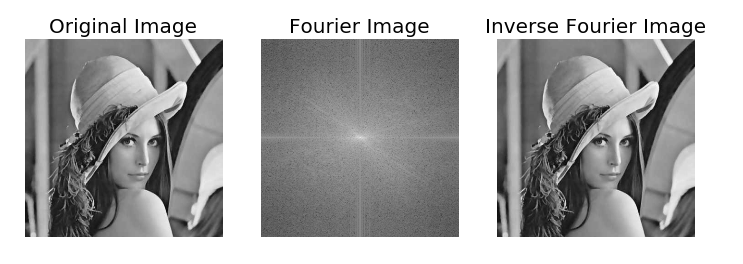# 四.OpenCV实现傅里叶变换

OpenCV 中相应的函数是cv2.dft()和用Numpy输出的结果一样，但是是双通道的。第一个通道是结果的实数部分，第二个通道是结果的虚数部分，并且输入图像要首先转换成 np.float32 格式。其函数原型如下所示：

dst = cv2.dft(src, dst=None, flags=None, nonzeroRows=None)

• src表示输入图像，需要通过np.float32转换格式
• dst表示输出图像，包括输出大小和尺寸
• flags表示转换标记，其中DFT _INVERSE执行反向一维或二维转换，而不是默认的正向转换；DFT _SCALE表示缩放结果，由阵列元素的数量除以它；DFT _ROWS执行正向或反向变换输入矩阵的每个单独的行，该标志可以同时转换多个矢量，并可用于减少开销以执行3D和更高维度的转换等；DFT _COMPLEX_OUTPUT执行1D或2D实数组的正向转换，这是最快的选择，默认功能；DFT _REAL_OUTPUT执行一维或二维复数阵列的逆变换，结果通常是相同大小的复数数组，但如果输入数组具有共轭复数对称性，则输出为真实数组
• nonzeroRows表示当参数不为零时，函数假定只有nonzeroRows输入数组的第一行（未设置）或者只有输出数组的第一个（设置）包含非零，因此函数可以处理其余的行更有效率，并节省一些时间；这种技术对计算阵列互相关或使用DFT卷积非常有用

cv2.magnitude(x, y)

• x表示浮点型X坐标值，即实部
• y表示浮点型Y坐标值，即虚部
最终输出结果为幅值，即：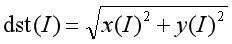``````# -*- coding: utf-8 -*-
import numpy as np
import cv2
from matplotlib import pyplot as plt

#读取图像

#傅里叶变换
dft = cv2.dft(np.float32(img), flags = cv2.DFT_COMPLEX_OUTPUT)

#将频谱低频从左上角移动至中心位置
dft_shift = np.fft.fftshift(dft)

#频谱图像双通道复数转换为0-255区间
result = 20*np.log(cv2.magnitude(dft_shift[:,:,0], dft_shift[:,:,1]))

#显示图像
plt.subplot(121), plt.imshow(img, cmap = 'gray')
plt.title('Input Image'), plt.xticks([]), plt.yticks([])
plt.subplot(122), plt.imshow(result, cmap = 'gray')
plt.title('Magnitude Spectrum'), plt.xticks([]), plt.yticks([])
plt.show()
``````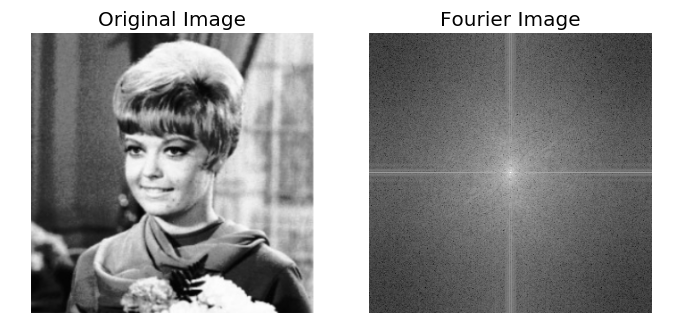# 五.OpenCV实现傅里叶逆变换

dst = cv2.idft(src[, dst[, flags[, nonzeroRows]]])

• src表示输入图像，包括实数或复数
• dst表示输出图像
• flags表示转换标记
• nonzeroRows表示要处理的dst行数，其余行的内容未定义（请参阅dft描述中的卷积示例）

``````# -*- coding: utf-8 -*-
import numpy as np
import cv2
from matplotlib import pyplot as plt

#读取图像

#傅里叶变换
dft = cv2.dft(np.float32(img), flags = cv2.DFT_COMPLEX_OUTPUT)
dftshift = np.fft.fftshift(dft)
res1= 20*np.log(cv2.magnitude(dftshift[:,:,0], dftshift[:,:,1]))

#傅里叶逆变换
ishift = np.fft.ifftshift(dftshift)
iimg = cv2.idft(ishift)
res2 = cv2.magnitude(iimg[:,:,0], iimg[:,:,1])

#显示图像
plt.subplot(131), plt.imshow(img, 'gray'), plt.title('Original Image')
plt.axis('off')
plt.subplot(132), plt.imshow(res1, 'gray'), plt.title('Fourier Image')
plt.axis('off')
plt.subplot(133), plt.imshow(res2, 'gray'), plt.title('Inverse Fourier Image')
plt.axis('off')
plt.show()
``````# 六.总结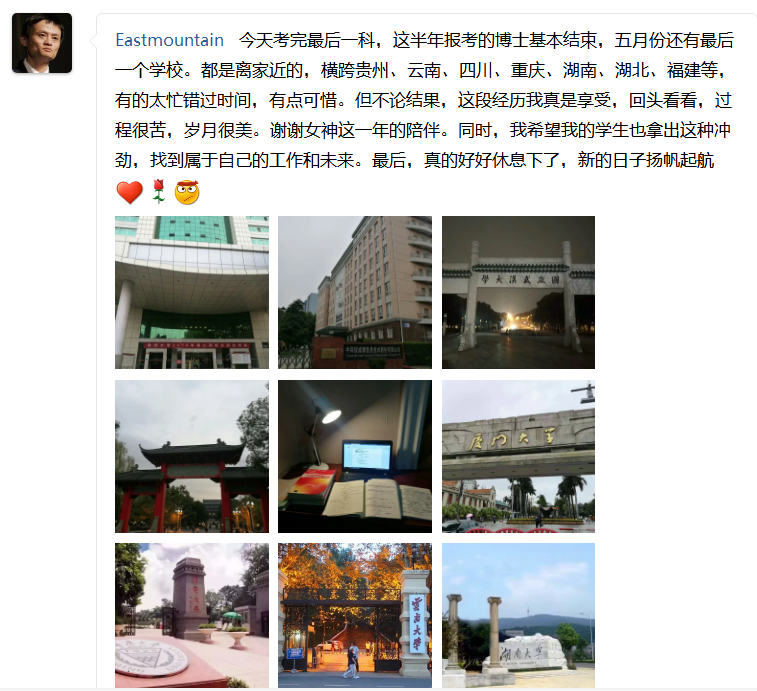（By：Eastmount 2019-04-23 周二下午6点写于花溪 https://blog.csdn.net/Eastmount )